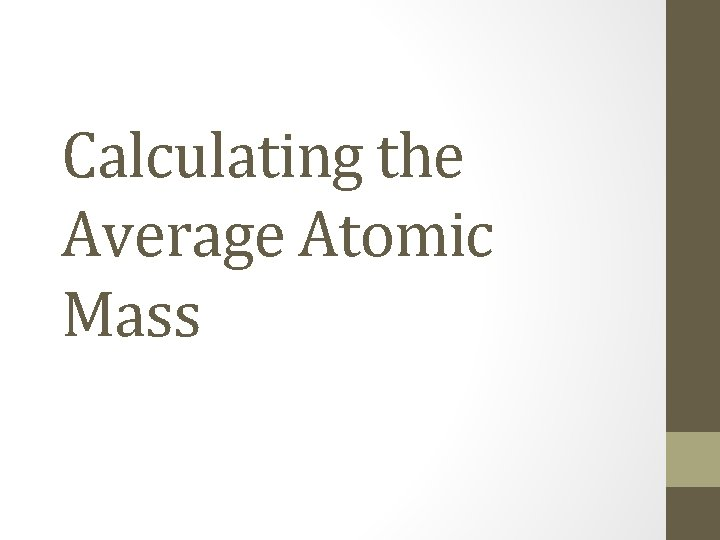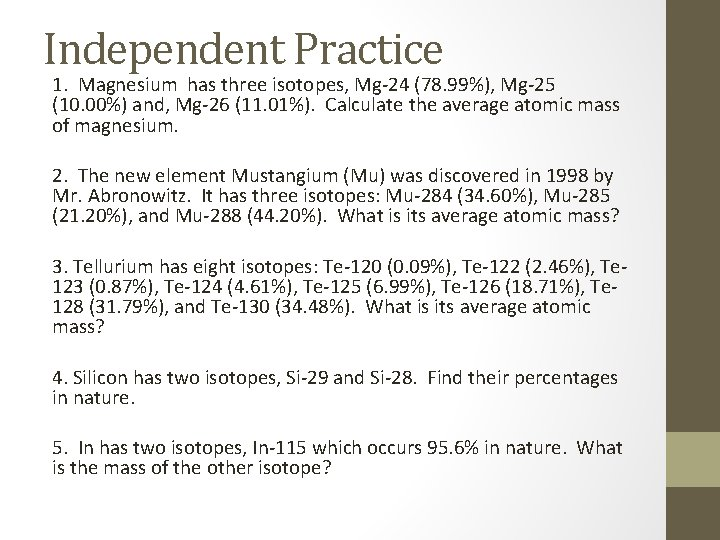# Calculating the Average Atomic Mass Steps for Calculating

• Slides: 3
Download presentationCalculating the Average Atomic MassSteps for Calculating Average Atomic Mass (When given percentages of each isotope and each isotopes mass) 1. Convert the percentages into decimals. (This percentage is known as its relative abundance or percent abundance). 2. Multiply the percentage of each isotope by its respective mass. 3. Add the numbers from step two together. Isotope Atomic Mass (u) Natural Abundance % Example: B-10 10. 02 amu 18. 83 B-11 11. 01 amu 81. 17 (percent abundance) (atomic mass) + (percent abundance) (atomic mass) (0. 1883) (10. 02 amu) + (0. 8117) (11. 01 amu) 1. 88677 + 8. 93682 = 10. 82359 amuIndependent Practice 1. Magnesium has three isotopes, Mg-24 (78. 99%), Mg-25 (10. 00%) and, Mg-26 (11. 01%). Calculate the average atomic mass of magnesium. 2. The new element Mustangium (Mu) was discovered in 1998 by Mr. Abronowitz. It has three isotopes: Mu-284 (34. 60%), Mu-285 (21. 20%), and Mu-288 (44. 20%). What is its average atomic mass? 3. Tellurium has eight isotopes: Te-120 (0. 09%), Te-122 (2. 46%), Te 123 (0. 87%), Te-124 (4. 61%), Te-125 (6. 99%), Te-126 (18. 71%), Te 128 (31. 79%), and Te-130 (34. 48%). What is its average atomic mass? 4. Silicon has two isotopes, Si-29 and Si-28. Find their percentages in nature. 5. In has two isotopes, In-115 which occurs 95. 6% in nature. What is the mass of the other isotope?